# Molar concentration facts for kids

Kids Encyclopedia Facts
(Redirected from Molarity)

Molar concentration, or molarity, or simply concentration, is a term in physical chemistry. It measures the concentration of a solution or mixture.

In chemistry, the molar concentration,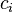$c_i$ is defined as the amount of a constituent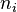$n_i$ (usually measured in moles – hence the name) divided by the volume of the mixture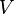$V$: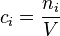$c_i = \frac {n_i}{V}$

The volume$V$ in the definition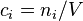$c_i = n_i/V$ refers to the volume of the solution, not the volume of the solvent. One litre of a solution usually contains either slightly more or slightly less than 1 litre of solvent because when a substance dissolves in a solvent it causes volume of liquid to increase or decrease.

The reciprocal quantity represents the dilution (volume) which can appear in Ostwald's law of dilution.Molar concentration Facts for Kids. Kiddle Encyclopedia.